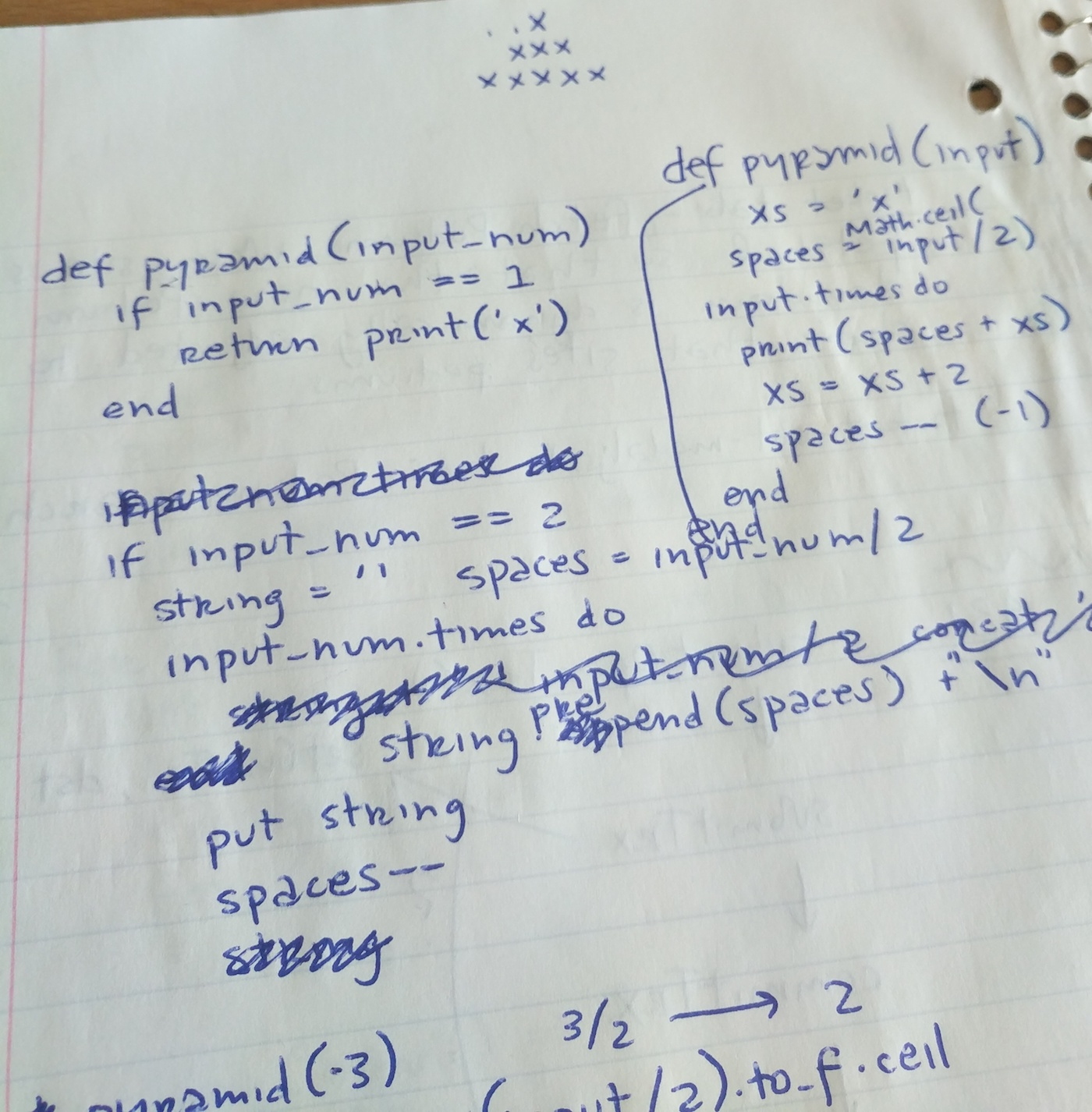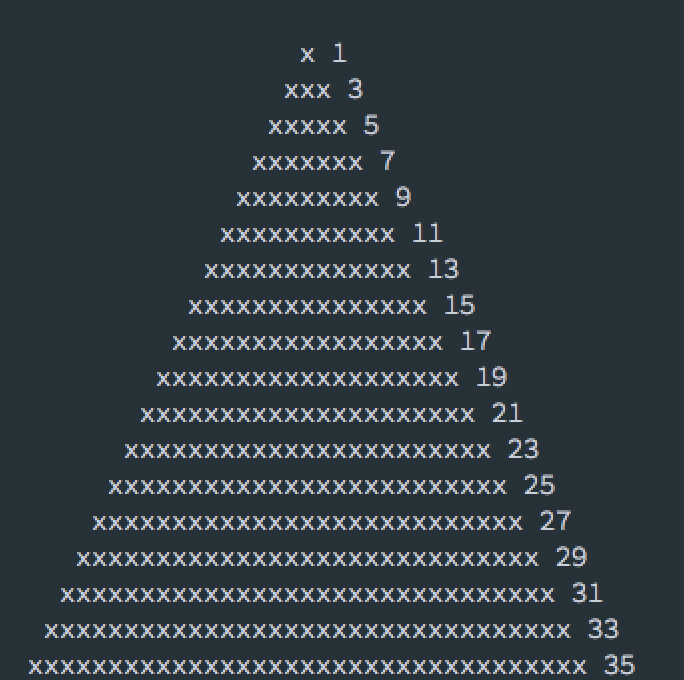## Pyramid of Doom - or - My Problem Solving Algorithm

Siggy Hinds · Jul 27, 2018

Problem Solving · Algorithms · Python · JavaScript

We recently had a new intern at Olio Apps and during the first couple days they were given a mini coding exercise to work through. By way of Friday afternoon banter, this was extended as a challenge to the rest of us devs to come up with our own solution. I wanted to write down my approach to solving this problem because it may be useful for some student, intern or Jr. dev preparing for an interview.

### The problem:

Write a program which asks the user for a number (n) and then prints out a pyramid shape of "x" characters with a height of n.

Not too bad, but where do you start? Disclaimer: People are going to have different approaches to problem solving along with different solutions. Below is mine. Is it the best? Probably not, but that's really not the point! The point is that I have a problem solving process which helps me get to an answer. If I play my cards right I'll show you my colleagues solutions at the end - I haven't seen them yet but I am sure there will be some differences.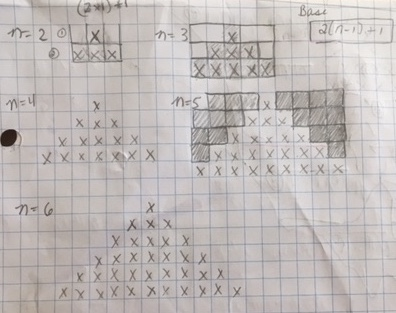First, because I am a visual person, I got out my paper and a pencil and started drawing pyramids of (n) size. Paper is the best place to try out different ideas make mistakes...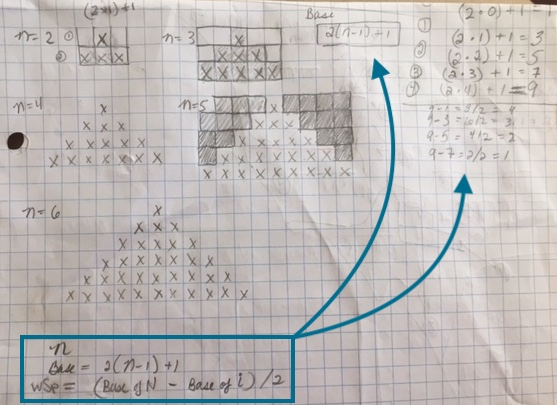Next, I looked for patterns which helped me come up with formulas for how many x's and spaces I should output for each row of the pyramid (Yay Math!)

This problem works well when looking from the bottom up. How many x's fill the base of the pyramid and what is the relationship of the number of x's and white space in the row above it. I found that the count of "x" at the base of the pyramid was 2(n-1) + 1. The count of "x" for any row (i) is the same 2(n-i) + 1. The total count of white spaces needed is the count of Xs at the base minus the count of X's ( base of n - base of i) / 2 (only the left side white space really counts.)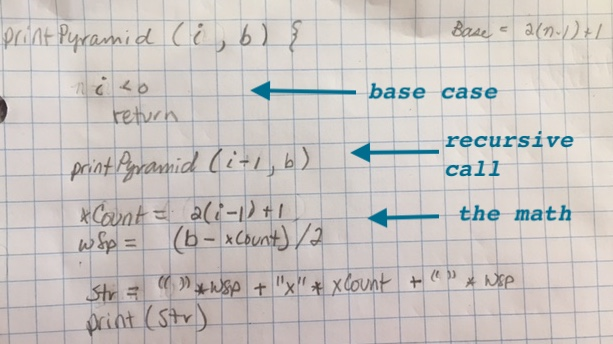Now that I have the math I started to work on the algorithm to use in my program. I could have done nested for loops but since I had already broken the problem up into sub problems it screamed recursion. I wrote this out on paper first too... this isn't perfect but it's a good start.

Next, I wrote the code. Ran it... found some minor errors I needed to tweak, and Voilá done! Here's my program in Python:

``````def printPyramid(i, b):
""" A recursive function that prints a pyramid of "X" characters
of i height to the screen

param1: i int, number of rows to print (height of pyramid)
param2: b int, the base or width of the pyramid

returns: void
"""

if(i < 0):
return

printPyramid(i-1, b)

xCount = 2 * (i-1) + 1
spCount = int((b - xCount ) / 2)

print(" " * spCount + "X" * xCount)

def main():
n = input("Enter a number ")
print('You entered', n)
base = 2 * (int(n)-1) + 1
printPyramid(int(n), base)

if __name__ == '__main__':
main()

``````

Here's the output...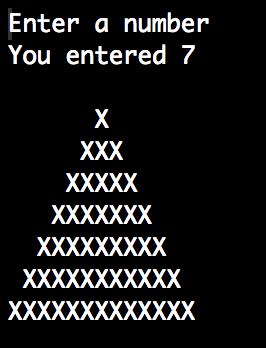## As promised here are my co-workers solutions:

After a few days we got together during lunch to demo our solutions. Some of us started on paper and others worked code out in the browser console. The most striking thing in the demo was that each of us had come up with a different solution and approach to solving the same problem.

Below are two solutions to this problem using an iterative approach in Javascript.

## Co-worker Solution #1

``````const buildPyramid = (levels) => {
let count = 1
const bricks = levels + levels
const protoPyramid = []

while (count < bricks) {
for (var j = 1; j < levels; j++) {
protoPyramid.push(".")
}

for (var i = 0; i < count; i++) {
protoPyramid.push("x")
}

for (var j = 1; j < levels; j++) {
protoPyramid.push(".")
}
protoPyramid.push("\n")
count += 2
levels -= 1
}

console.log(protoPyramid.join(""))
}
``````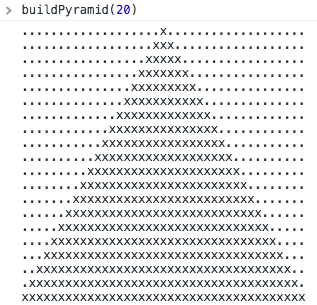## Co-worker Solution #2

``````const pyramid = (input) => {
let exes = 1
let spaces = input

for (let i = 0; spaces > 0; i++) {
console.log(" ".repeat(spaces), "x".repeat(exes), exes)
exes += 2
spaces -= 1
}
}
pyramid(process.argv)
``````